# RD Sharma Solutions for Class 9 Maths Chapter 17 Construction Exercise 17.1

Exercise 17.1 of RD Sharma textbook is based on the topic Construction, and students will need to solve problems based on it. In this exercise, students will learn how to draw a perpendicular bisector for a given line segment. RD Sharma study material helps students to score good marks in the examination. Solution for each question is given according to the exercises in the RD Sharma Maths book for Class 9. Check the detailed RD Sharma Solutions for Class 9 Chapter 17 – Construction below.

## Download PDF of RD Sharma Solutions for Class 9 Maths Chapter 17 Construction Exercise 17.1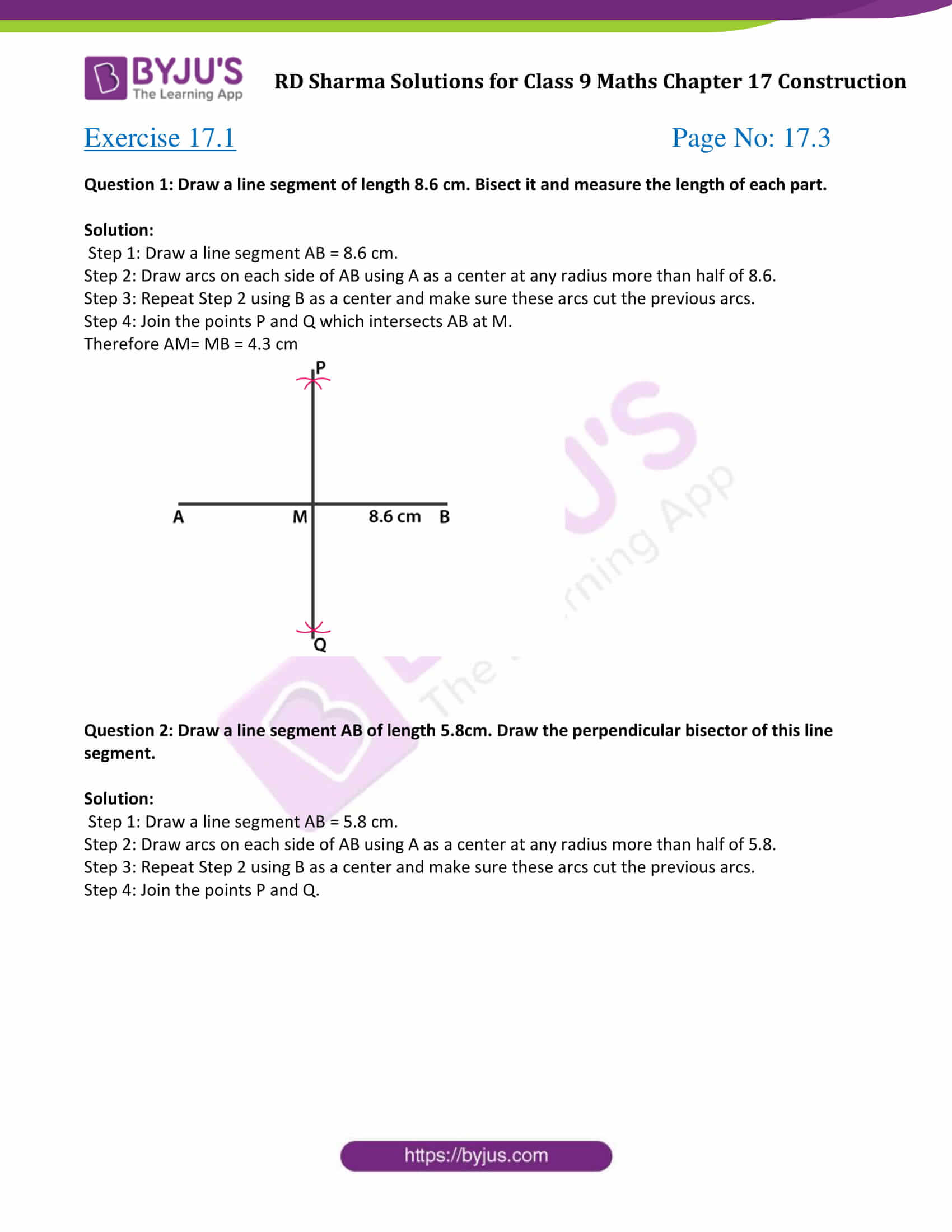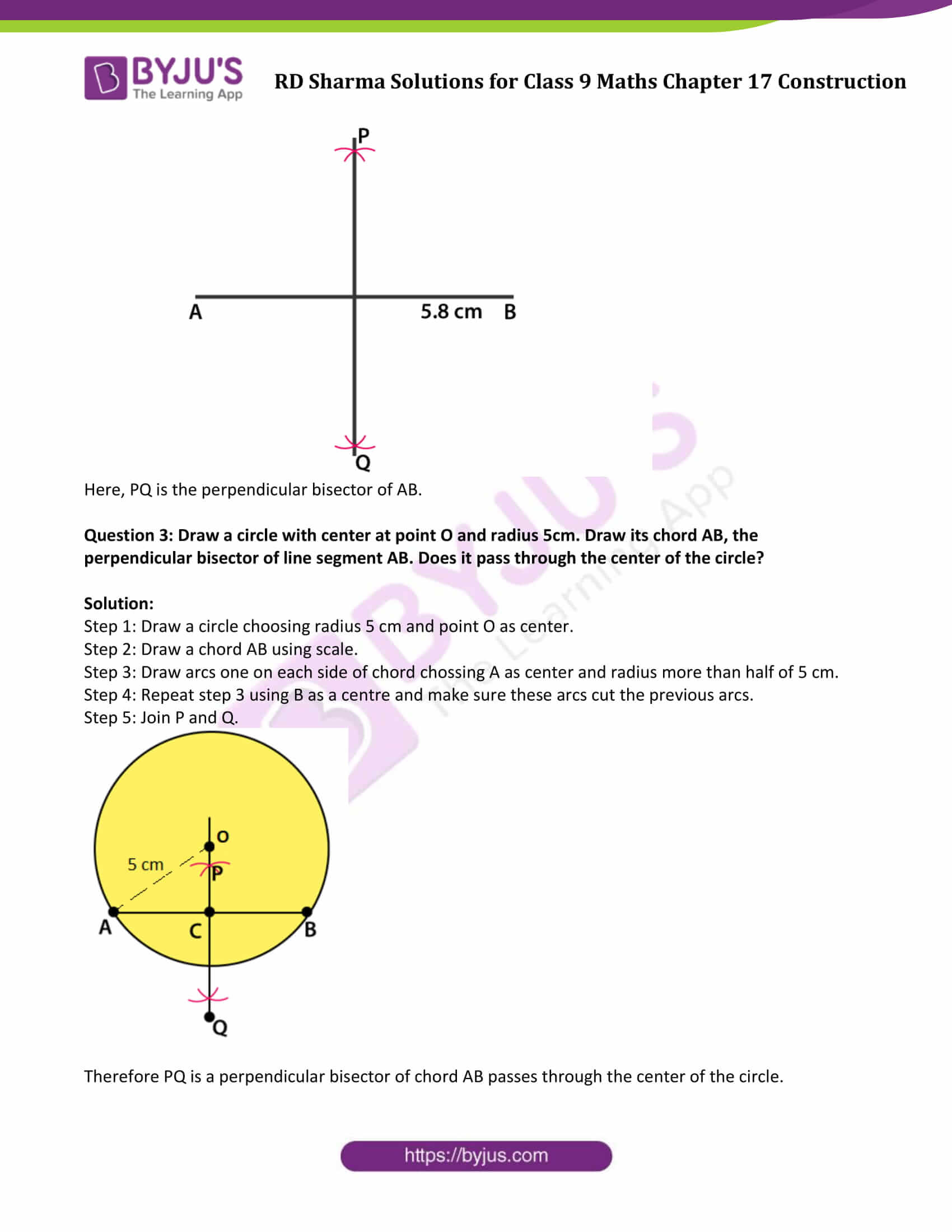### Access Answers to Maths RD Sharma Solutions for Class 9 Chapter 17 Construction Exercise 17.1 Page number 17.3

Question 1: Draw a line segment of length 8.6 cm. Bisect it and measure the length of each part.

Solution:

Step 1: Draw a line segment AB = 8.6 cm.

Step 2: Draw arcs on each side of AB using A as a center at any radius more than half of 8.6.

Step 3: Repeat Step 2 using B as a center and make sure these arcs cut the previous arcs.

Step 4: Join the points P and Q which intersects AB at M.

Therefore AM= MB = 4.3 cm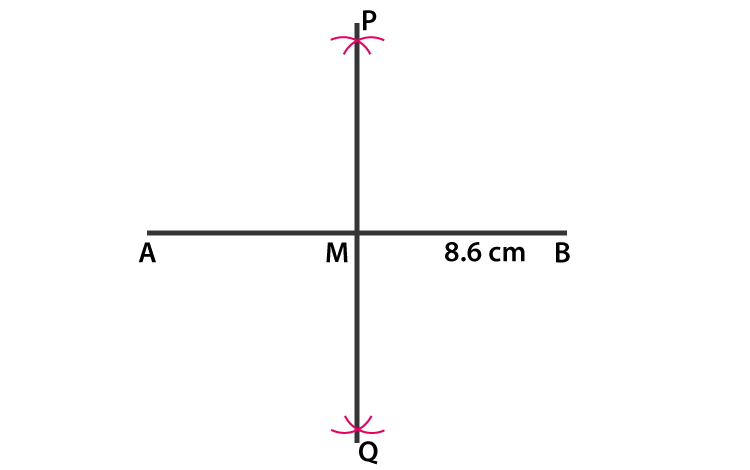Question 2: Draw a line segment AB of length 5.8cm. Draw the perpendicular bisector of this line segment.

Solution:

Step 1: Draw a line segment AB = 5.8 cm.

Step 2: Draw arcs on each side of AB using A as a center at any radius more than half of 5.8.

Step 3: Repeat Step 2 using B as a center and make sure these arcs cut the previous arcs.

Step 4: Join the points P and Q.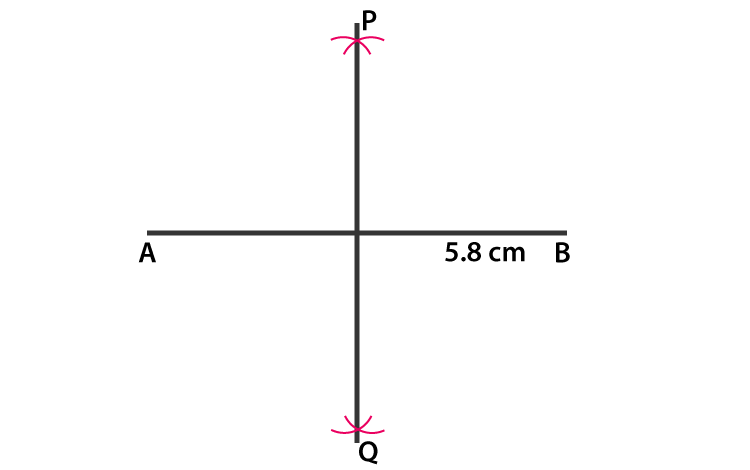Here, PQ is the perpendicular bisector of AB.

Question 3: Draw a circle with center at point O and radius 5cm. Draw its chord AB, the perpendicular bisector of line segment AB. Does it pass through the center of the circle?

Solution:

Step 1: Draw a circle choosing radius 5 cm and point O as center.

Step 2: Draw a chord AB using scale.

Step 3: Draw arcs one on each side of chord chossing A as center and radius more than half of 5 cm.

Step 4: Repeat step 3 using B as a centre and make sure these arcs cut the previous arcs.

Step 5: Join P and Q.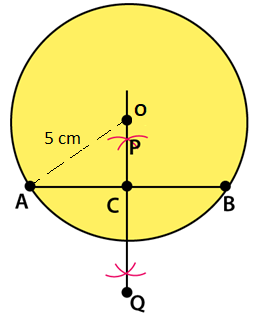Therefore PQ is a perpendicular bisector of chord AB passes through the center of the circle.

## RD Sharma Solutions for Class 9 Maths Chapter 17 Construction Exercise 17.1

Class 9 Maths Chapter 17 Construction Exercise 17.1 is based on the topic – Construction of Bisector of a Line Segment. Students can access all the RD Sharma solutions in the exact sequence as in the class 9 textbook.